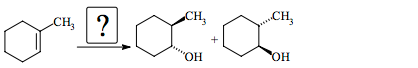# Problem: Provide the following when a product is given. If an organic reactant is missing, supply a structural formula; if an inorganic reactant (reagent) or catalyst is missing, simply give a formula.

###### FREE Expert Solution
88% (338 ratings)
###### Problem Details

Provide the following when a product is given. If an organic reactant is missing, supply a structural formula; if an inorganic reactant (reagent) or catalyst is missing, simply give a formula.Frequently Asked Questions

What scientific concept do you need to know in order to solve this problem?

Our tutors have indicated that to solve this problem you will need to apply the Hydroboration concept. You can view video lessons to learn Hydroboration. Or if you need more Hydroboration practice, you can also practice Hydroboration practice problems.

What is the difficulty of this problem?

Our tutors rated the difficulty ofProvide the following when a product is given. If an organic...as medium difficulty.

How long does this problem take to solve?

Our expert Organic tutor, Jonathan took 1 minute and 58 seconds to solve this problem. You can follow their steps in the video explanation above.

What professor is this problem relevant for?

Based on our data, we think this problem is relevant for Professor Laird's class at IOWA.